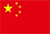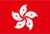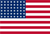• 下载App

扫码立即下载

+

• 首页
• 行情
• 资讯
• 牛牛圈
• 课堂
• 帮助中心
• 关于
• 语言
•••EN

# Is There An Opportunity With Dominion Energy, Inc.'s (NYSE:D) 48% Undervaluation?

Simply Wall St ·  02/06 20:57

Today we'll do a simple run through of a valuation method used to estimate the attractiveness of Dominion Energy, Inc. (NYSE:D) as an investment opportunity by taking the expected future cash flows and discounting them to today's value. Our analysis will employ the Discounted Cash Flow (DCF) model. Don't get put off by the jargon, the math behind it is actually quite straightforward.

We would caution that there are many ways of valuing a company and, like the DCF, each technique has advantages and disadvantages in certain scenarios. For those who are keen learners of equity analysis, the Simply Wall St analysis model here may be something of interest to you.

See our latest analysis for Dominion Energy

## Step By Step Through The Calculation

We are going to use a two-stage DCF model, which, as the name states, takes into account two stages of growth. The first stage is generally a higher growth period which levels off heading towards the terminal value, captured in the second 'steady growth' period. To start off with, we need to estimate the next ten years of cash flows. Where possible we use analyst estimates, but when these aren't available we extrapolate the previous free cash flow (FCF) from the last estimate or reported value. We assume companies with shrinking free cash flow will slow their rate of shrinkage, and that companies with growing free cash flow will see their growth rate slow, over this period. We do this to reflect that growth tends to slow more in the early years than it does in later years.

A DCF is all about the idea that a dollar in the future is less valuable than a dollar today, so we discount the value of these future cash flows to their estimated value in today's dollars:

#### 10-year free cash flow (FCF) forecast

 2023 2024 2025 2026 2027 2028 2029 2030 2031 2032 Levered FCF (\$, Millions) -US\$2.79b -US\$2.39b -US\$765.0m US\$1.70b US\$2.65b US\$3.71b US\$4.76b US\$5.74b US\$6.60b US\$7.34b Growth Rate Estimate Source Analyst x3 Analyst x2 Analyst x1 Analyst x1 Est @ 55.88% Est @ 39.74% Est @ 28.44% Est @ 20.53% Est @ 14.99% Est @ 11.11% Present Value (\$, Millions) Discounted @ 6.8% -US\$2.6k -US\$2.1k -US\$628 US\$1.3k US\$1.9k US\$2.5k US\$3.0k US\$3.4k US\$3.6k US\$3.8k

("Est" = FCF growth rate estimated by Simply Wall St)
Present Value of 10-year Cash Flow (PVCF) = US\$14b

After calculating the present value of future cash flows in the initial 10-year period, we need to calculate the Terminal Value, which accounts for all future cash flows beyond the first stage. For a number of reasons a very conservative growth rate is used that cannot exceed that of a country's GDP growth. In this case we have used the 5-year average of the 10-year government bond yield (2.1%) to estimate future growth. In the same way as with the 10-year 'growth' period, we discount future cash flows to today's value, using a cost of equity of 6.8%.

Terminal Value (TV)= FCF2032 × (1 + g) ÷ (r – g) = US\$7.3b× (1 + 2.1%) ÷ (6.8%– 2.1%) = US\$158b

Present Value of Terminal Value (PVTV)= TV / (1 + r)10= US\$158b÷ ( 1 + 6.8%)10= US\$81b

The total value is the sum of cash flows for the next ten years plus the discounted terminal value, which results in the Total Equity Value, which in this case is US\$96b. The last step is to then divide the equity value by the number of shares outstanding. Compared to the current share price of US\$60.3, the company appears quite undervalued at a 48% discount to where the stock price trades currently. Valuations are imprecise instruments though, rather like a telescope - move a few degrees and end up in a different galaxy. Do keep this in mind.

## Important Assumptions

The calculation above is very dependent on two assumptions. The first is the discount rate and the other is the cash flows. Part of investing is coming up with your own evaluation of a company's future performance, so try the calculation yourself and check your own assumptions. The DCF also does not consider the possible cyclicality of an industry, or a company's future capital requirements, so it does not give a full picture of a company's potential performance. Given that we are looking at Dominion Energy as potential shareholders, the cost of equity is used as the discount rate, rather than the cost of capital (or weighted average cost of capital, WACC) which accounts for debt. In this calculation we've used 6.8%, which is based on a levered beta of 0.800. Beta is a measure of a stock's volatility, compared to the market as a whole. We get our beta from the industry average beta of globally comparable companies, with an imposed limit between 0.8 and 2.0, which is a reasonable range for a stable business.

### SWOT Analysis for Dominion Energy

Strength
• Debt is well covered by earnings.
• Dividend is in the top 25% of dividend payers in the market.
• Dividend information for D.
Weakness
• Earnings declined over the past year.
• Shareholders have been diluted in the past year.
Opportunity
• Annual earnings are forecast to grow for the next 4 years.
• Trading below our estimate of fair value by more than 20%.
Threat
• Debt is not well covered by operating cash flow.
• Dividends are not covered by earnings.
• Annual earnings are forecast to grow slower than the American market.
• Is D well equipped to handle threats?